java基础
1131 浏览 | 2020-11-30 | 阅读时间: 约 26 分钟 | 分类: JAVA | 标签: JAVA,JAVA基础

# 1.常用快捷键 快捷输入

## 1.1常用快捷键

• ctrl+shift+L 格式化代码格式
• alt+Inster 插入代码（get，set方法）
• alt+entrer 导入包，自动修正代码
• ctr+/ 一行注释
• ctrl+shift+/ 多行注释
• ctrl+shift+方向键上下 移动当前行代码
• ctrl+D 复制当前行到下一行
• ctrl+Y 删除光标所在行
• alt+/ 补全代码（自己修改的）
• Ctrl + Alt + T 对选中的代码弹出环绕选项弹出层

## 1.2 快捷输入

### 1.2.1 psvm(快速输入main方法)

``public static void main(String[] args) {}``

### 1.2.2 sout(快速输入输出方法)

``System.out.println();``

### 1.2.4 [要循环的对象].fori

``````        // 打印所有数组元素 快捷写法 数组名称.fori (mylist.fori)
for (int i = 0; i < myList.length; i++) {
System.out.println(myList[i]);
}``````

### 1.2.5 for—each增强型循环

``````/*JDK 1.5 引进了一种新的循环类型，被称为 For-Each 循环或者加强型循环，它能在不用下标的情况下遍历数组。

for(type element: array)
{
System.out.println(element);
}``````

# 2. 【常量、变量】

## 2.1 常量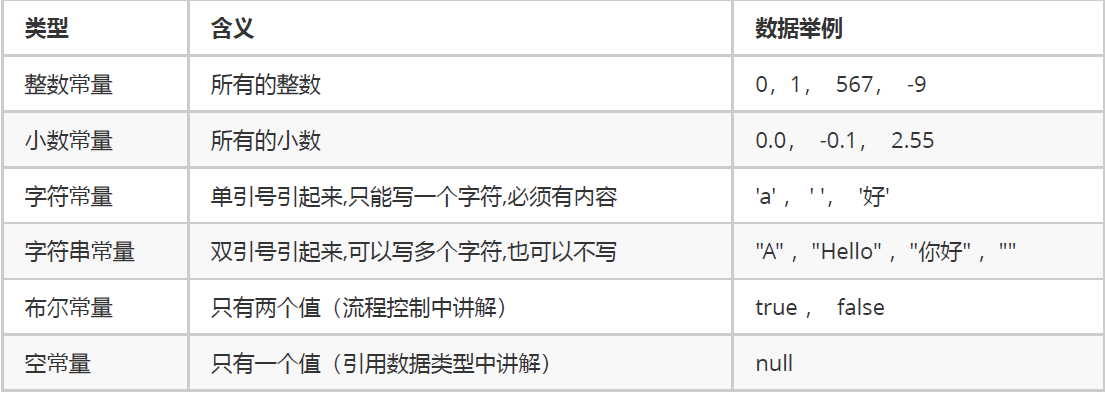``````public class ConstantDemo {
public static void main(String[] args){
//输出整数常量
System.out.println(123);
//输出小数常量
System.out.println(0.125);
//输出字符常量
System.out.println('A');
//输出布尔常量
System.out.println(true);
//输出字符串常量
System.out.println("你好Java");
}``````

## 2.2 变量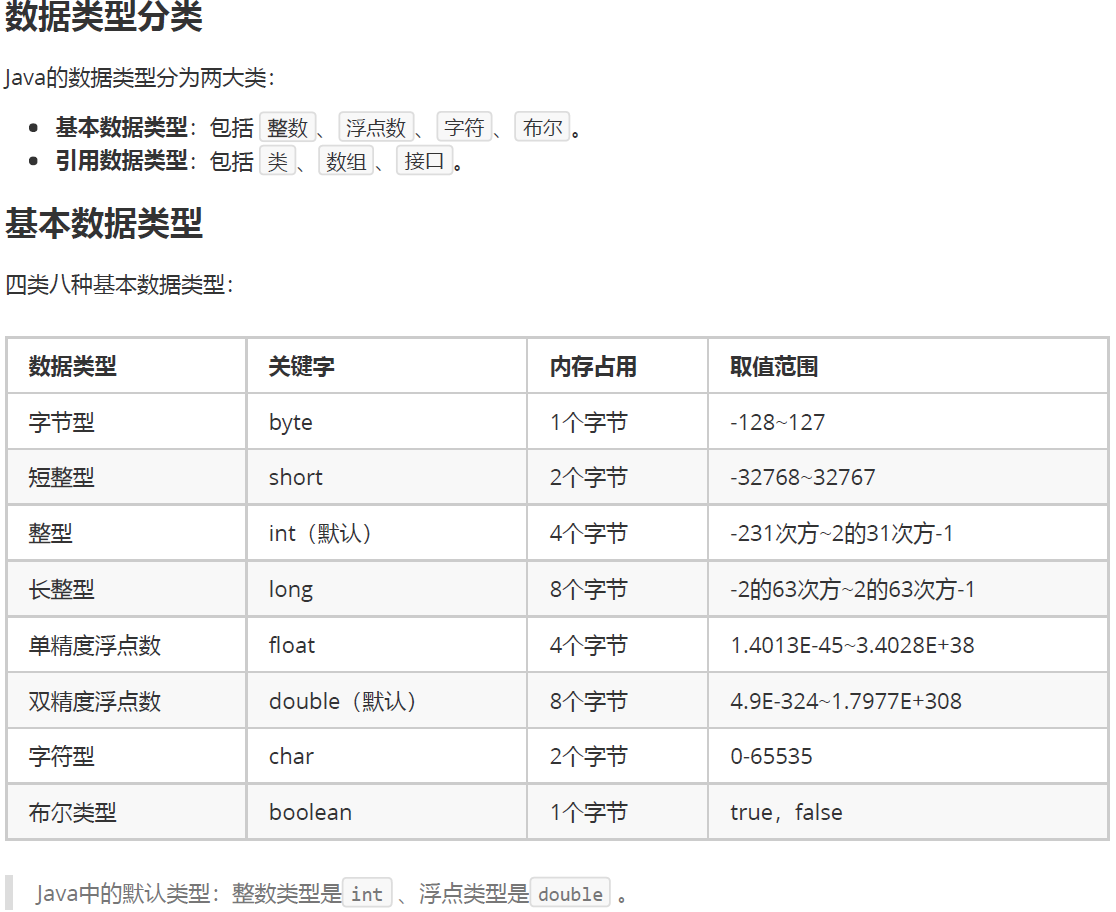``````public class Variable {
public static void main(String[] args){
//定义字节型变量
byte b = 100;
System.out.println(b);
//定义短整型变量
short s = 1000;
System.out.println(s);
//定义整型变量
int i = 123456;
System.out.println(i);
//定义长整型变量
long l = 12345678900L;
System.out.println(l);
//定义单精度浮点型变量
float f = 5.5F;
System.out.println(f);
//定义双精度浮点型变量
double d = 8.5;
System.out.println(d);
//定义布尔型变量
boolean bool = false;
System.out.println(bool);
//定义字符型变量
char c = 'A';
System.out.println(c);
}
}``````

# 3. 【数据类型转换、运算符】

## 3.1数据类型

### 3.1.1 自动转换

byte 类型内存占有1个字节，在和int 类型运算时会提升为int 类型 ，自动补充3个字节，因此计算后的结果还是int 类型。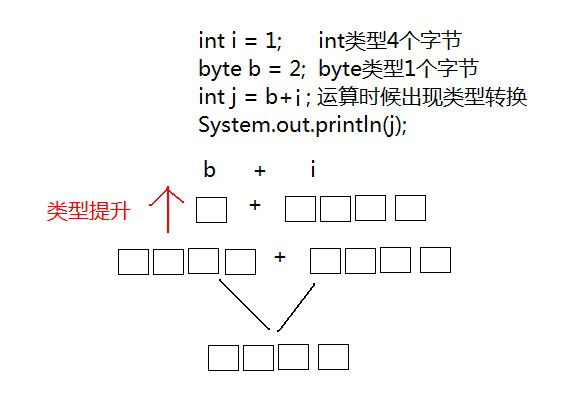``````public static void main(String[] args) {
int i = 1;
double d = 2.5;
//int类型和double类型运算，结果是double类型
//int类型会提升为double类型
double e = d+i;
System.out.println(e);
}``````

``byte、short、char‐‐>int‐‐>long‐‐>float‐‐>double``

### 3.1.2 强制转换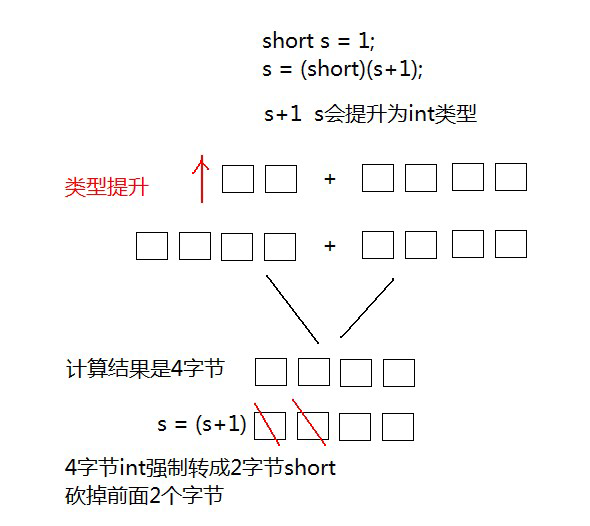int 强制转成short 砍掉2个字节，可能造成数据丢失。

``````// 定义s为short范围内最大值
short s = 32767;
// 运算后，强制转换，砍掉2个字节后会出现不确定的结果
s = (short)(s + 10);``````

048
957
A65
Z90
a97
z122

## 3.2 运算符

### 3.2.1 算数运算符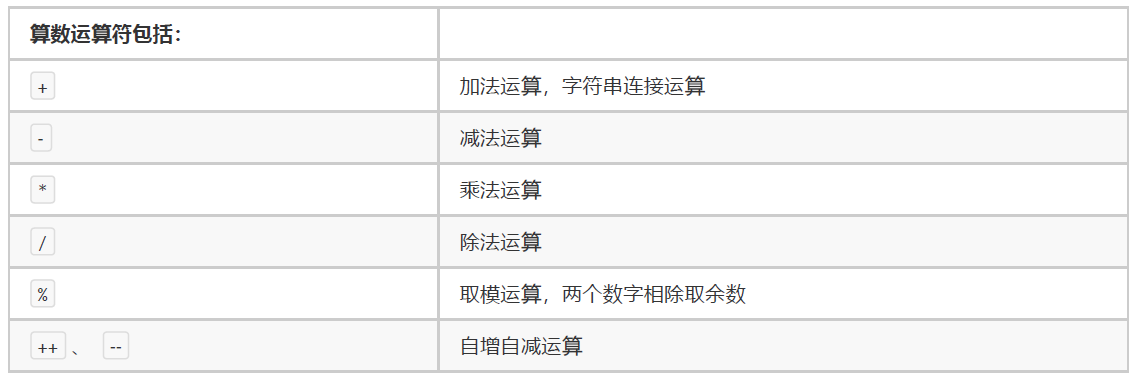### 3.2.2 赋值运算符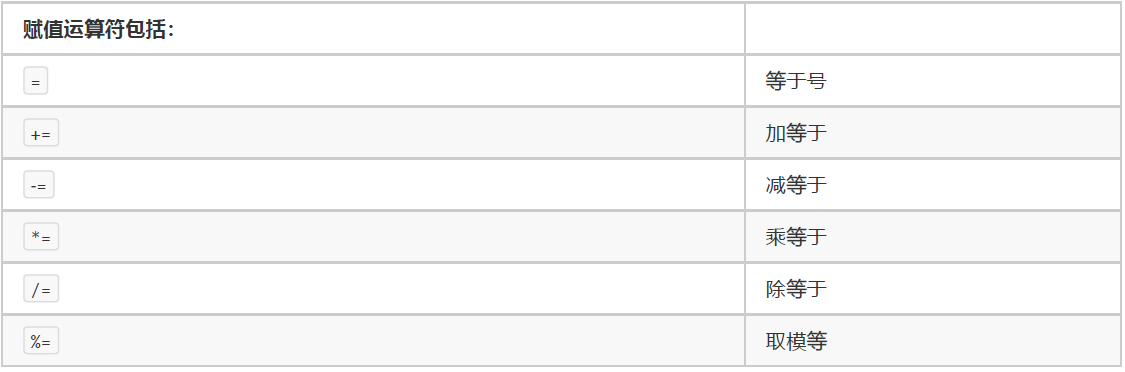### 3.2.3 比较运算符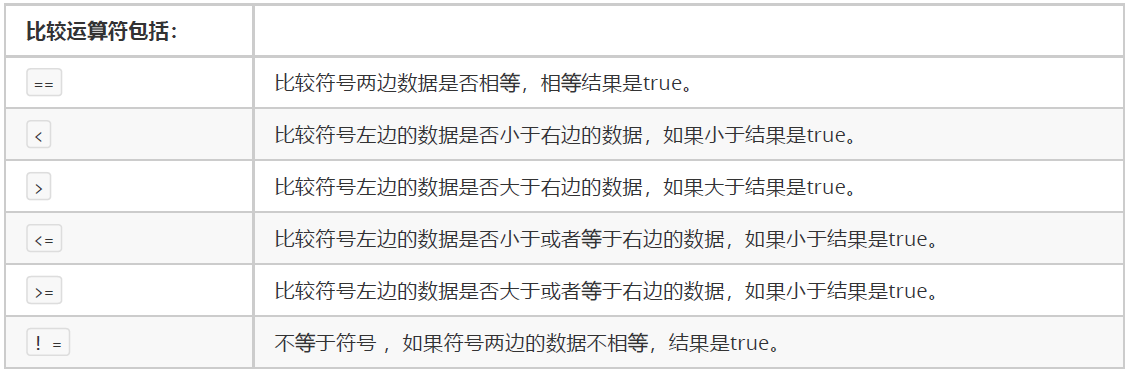### 3.2.4 逻辑运算符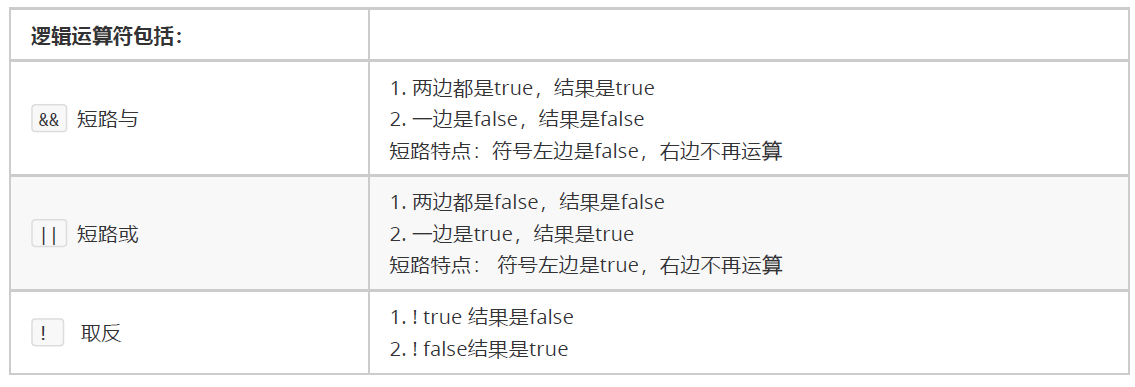### 3.2.5 三元运算符

``````//数据类型 变量名 = 布尔类型表达式？结果1：结果2
//布尔类型表达式结果是true，三元运算符整体结果为结果1，赋值给变量。
//布尔类型表达式结果是false，三元运算符整体结果为结果2，赋值给变量。
public static void main(String[] args) {
int i = (1==2 ? 100 : 200);
System.out.println(i);//200
int j = (3<=4 ? 500 : 600);
System.out.println(j);//500
}``````

### 3.2.6 位运算符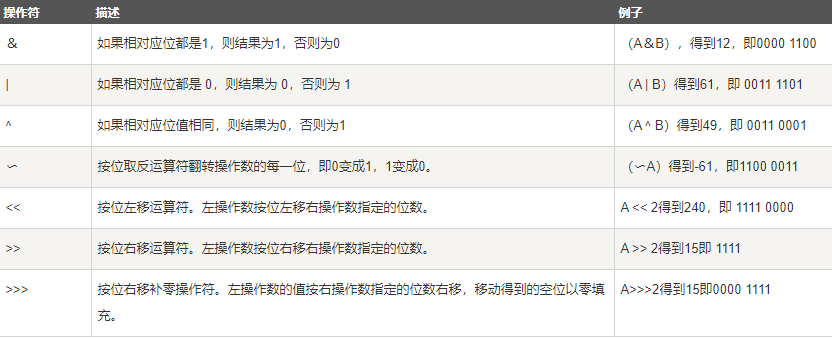### 3.2.7 instanceof运算符 (多用于类的上下转型)

``````class Vehicle {}

public class Car extends Vehicle {
public static void main(String[] args){
Vehicle a = new Car();
boolean result =  a instanceof Car;
System.out.println( result);
}
}``````
``````/*

*/
public class Demo02Instanceof {

public static void main(String[] args) {
Animal animal = new Dog(); // 本来是一只狗
animal.eat(); // 狗吃SHIT

// 如果希望掉用子类特有方法，需要向下转型
// 判断一下父类引用animal本来是不是Dog
if (animal instanceof Dog) {
Dog dog = (Dog) animal;
dog.watchHouse();
}
// 判断一下animal本来是不是Cat
if (animal instanceof Cat) {
Cat cat = (Cat) animal;
cat.catchMouse();
}

giveMeAPet(new Dog());
}

public static void giveMeAPet(Animal animal) {
if (animal instanceof Dog) {
Dog dog = (Dog) animal;
dog.watchHouse();
}
if (animal instanceof Cat) {
Cat cat = (Cat) animal;
cat.catchMouse();
}
}

}
``````

## 3.3 运算符的优先级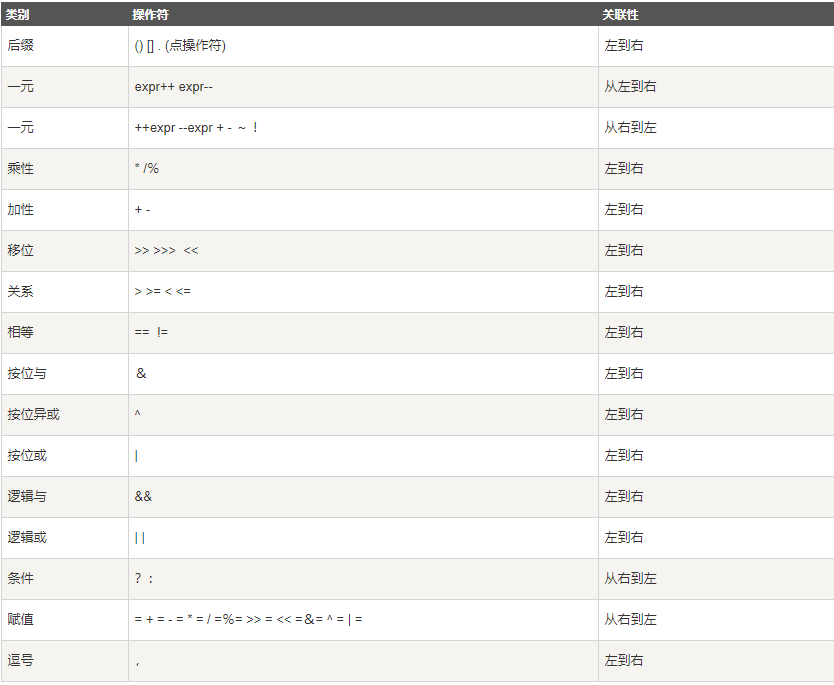# 4. 【数组】

## 4.1数组的定义

### 4.1.1 方式一

• 格式

``数组存储的数据类型[] 数组名字 = new 数组存储的数据类型[长度];``
• 数组定义格式详解：

• 数组存储的数据类型： 创建的数组容器可以存储什么数据类型。
• [] : 表示数组。
• 数组名字：为定义的数组起个变量名，满足标识符规范，可以使用名字操作数组。
• new：关键字，创建数组使用的关键字。
• 数组存储的数据类型： 创建的数组容器可以存储什么数据类型。
• [长度]：数组的长度，表示数组容器中可以存储多少个元素。
• 注意：数组有定长特性，长度一旦指定，不可更改。

• 和水杯道理相同，买了一个2升的水杯，总容量就是2升，不能多也不能少。
• 举例：

``int[] arr = new int;``

### 4.1.2 方式二

• 格式

``数据类型[] 数组名 = new 数据类型[]{元素1,元素2,元素3...};``
• 举例：

``int[] arr = new int[]{1,2,3,4,5};``

### 4.1.3 方式三

• 格式
``数据类型[] 数组名 = {元素1,元素2,元素3...};``
• 举例：
``int[] arr = {1,2,3,4,5};``

## 4.2 数组访问

``````public class Array {
//数组大小
static final int size = 10;

public static void main(String[] args) {
//定义数组
double[] myList = new double[size];
myList = 5.6;
myList = 4.5;
myList = 3.3;
myList = 13.2;
myList = 4.0;
myList = 34.33;
myList = 34.0;
myList = 45.45;
myList = 99.993;
myList = 11123;
double sum = 0;
//System.out.println(Arrays.toString(myList));
for (int i = 0; i < 10; i++) {
sum += myList[i];
}
//        直接打印数组名称得到的是数组内存哈希值（16进制）
System.out.println("直接打印数组名称"+myList);
System.out.println("总和为：" + sum);

// 打印所有数组元素 快捷写法 数组名称.fori (mylist.fori)
for (int i = 0; i < myList.length; i++) {
System.out.println(myList[i]);
}

// 查找最大元素
double max = myList;
for (int i = 1; i < myList.length; i++) {
if (max < myList[i]) {
max = myList[i];
}
}
System.out.println("max is " + max);
/*
JDK 1.5 引进了一种新的循环类型，被称为 For-Each 循环或者加强型循环，它能在不使用下标的情况下遍历数组。

for(type element: array)
{
System.out.println(element);
}
*/
for (double sum1 : myList) {
System.out.println(sum1);
}
//数组作为函数的参数
printArray(myList);
//数组作为函数的返回值
printArray(reverse(myList));
//排序升序
Arrays.sort(myList);
printArray(myList);
}

//数组作为函数的参数
public static void printArray(double[] array) {
for (double sum : array) {
System.out.println("printArray " + sum);
}
}

//数组作为函数的返回值
public static double[] reverse(double[] array) {

for (int i = 0, j = array.length - 1; i < array.length / 2; i++, j--) {
//注意数组下表 result.length=10 数组下表0开始所以减1
//注意i<array.length/2 下标走到一半就交换完了
double ex = array[i];
array[i] = array[j];
array[j] = ex;
}
return array;
}
}``````

# 5.【类与对象、封装、构造方法】

java been规范

``````public class ClassName{
//成员变量
//构造方法
//无参构造方法【必须】
//有参构造方法【建议】
//成员方法
//getXxx()
//setXxx()
}``````
``````public class Student {
//成员变量
private String name;
private int age;
//构造方法
public Student() {}
public Student(String name,int age) {
this.name = name;
this.age = age;
}
//成员方法
publicvoid setName(String name) {
this.name = name;
}
public String getName() {
return name;
}
publicvoid setAge(int age) {
this.age = age;
}
publicint getAge() {
return age;
}
}``````

# 6.【Scanner类、Random类、ArrayList类】

## 6.1 Scanner类(Scanner(System.in).next())

``````package com.scanner;

import java.util.Scanner;

/*1.导包
* 2.创建
* 3.使用*/
public class TextScanner {
public static void main(String[] args) {
Scanner sc= new Scanner(System.in);
System.out.println("输入数字1：");
int a=sc.nextInt();//获取数字
System.out.println("输入数字2：");
int b=sc.nextInt();
System.out.println("和为："+(a+b));

System.out.println("输入数字1：");
a=sc.nextInt();//获取数字
System.out.println("输入数字2：");
b=sc.nextInt();
System.out.println("输入数字2：");
int c=sc.nextInt();
int max=a>b?(a>c?a:c):(b>c?b:c);
System.out.println("最大值为："+max);

/*        System.out.println(sc.nextInt());//获取数字
System.out.println("输入字符串");
System.out.println(sc.next());//获取字符串
String str=new String();
str=new Scanner(System.in).next()//匿名使用
*/
}
}
``````

## 6.2 Random类(Random().nextInt();)

``````package com.random;

import java.util.Random;
import java.util.Scanner;

/*1.导包
* 2.创建
* 3.使用*/
public class TextRandom {
public static void main(String[] args) {
System.out.println("输入最大值：");
//Scanner sc = new Scanner(System.in);
int a = new Scanner(System.in).nextInt();//匿名对象 只用一次
//Random r = new Random();
int num=new Random().nextInt(a)+1;//匿名对象 只用一次

do {
System.out.println("你猜的数字：");
int b = new Scanner(System.in).nextInt();//匿名对象 只用一次
if (b==num){
System.out.println("猜对了！");
break;
}else if (b<num){
System.out.println("小了！");
}else if (b>num){
System.out.println("大了！");
}
else {
System.out.println("猜错了！");
}
}while (true);

}
}``````

## 6.3 ArrayList类(ArrayList<泛型>，add,get,size,remove)

### 6.3.1 对象数组

``````package com.array;

import java.util.ArrayList;
import java.util.Random;

/*数组长度不可变；
* ArrayList<E> ；<E>代表泛型 也即使集合中的所有元素 全都是统一的类型 只能是引用型不能是基本型
* 对于集合来说直接打印的是内容不是地址值
* */
public class ArrayListText {
public static void main(String[] args) {
ArrayList<String> list = new ArrayList<>();
System.out.println(list);
//add方法添加数据
list.add("Msy");
System.out.println(list);
list.add("Msy1");
list.add("Msy2");
list.add("Msy3");
System.out.println(list);
System.out.println(list.size());

//常用方法get；
System.out.println(list.get(2));
//常用方法renmove
System.out.println(list.remove(2));//remove 返回值是<Type>
System.out.println(list);
//常用方法size
System.out.println(list.size());

//集合遍历
for (int i = 0; i < list.size(); i++) {
System.out.println(list.get(i));
}
//增强循环
for (String str : list
) {
System.out.println(str);

}

//如果集合要存储基本类型就要使用基本类型的包装类
//        Integer;Character;Boolean;Long;Double:Short;Float;Byte;

ArrayList<Integer> list1 = new ArrayList<>();
list1.add(1000000000);
System.out.println(list1.get(0));

//生成1-33随机数放入集合中 并且遍历
ArrayList<Integer> list2 = new ArrayList<>();
for (int i = 0; i < 20; i++) {
int a = new Random().nextInt(33) + 1;
list2.add(a);
}
for (int i = 0; i < list2.size(); i++) {
System.out.println(list2.get(i));
}
for (int a : list2
) {
System.out.println(a);
}

printfArrayList(list2);

ArrayList<Integer> oushu = oushuArrayList(list2);
for (int i = 0; i < oushu.size(); i++) {
System.out.println(oushu.get(i));
}
}

//定义以指定格式打印集合的方法(ArrayList类型作为参数)，使用{}扩起集合，使用@分隔每个元素。格式参照 {元素
//@元素@元素}。
public static void printfArrayList(ArrayList<Integer> list) {
System.out.print("{");
for (int i = 0; i < list.size(); i++) {
System.out.print(list.get(i));
if (i == list.size() - 1) {
System.out.println("}");
} else {
System.out.print("@");
}

}
}

//定义获取所有偶数元素集合的方法(ArrayList类型作为返回值)
public static ArrayList<Integer> oushuArrayList(ArrayList<Integer> list) {
ArrayList<Integer> list1 = new ArrayList<>();
for (int i = 0; i < list.size(); i++) {
if (list.get(i) % 2 == 0) {
list1.add(list.get(i));
}
}
return list1;
}
}
``````

# 7.【String类、static、Arrays类、Math类】

## 7.1 String类

### 7.1.1判断功能方法(equals)

``````package com.string;
//public boolean equals (Object anObject) ：将此字符串与指定对象进行比较。
//public boolean equalsIgnoreCase (String anotherString) ：将此字符串与指定对象进行比较，忽略大小写。
public class StringEquals {
public static void main(String[] args) {
/*==是进行对象地址值比较，要对字符串内容进行比较 可以用两个方法*/
//对于基本类型来说==是对数值进行比较
//对于引用类型来说==是对地址值进行比较

//equals 注意常量在前
String str1 = "hello";
//String str1= new String; str1="hello"; 等价上句 String是个类 str1是类所创建的对象
String str2 = "hello";
char[] charArray = {'h', 'e', 'l', 'l', 'o'};
String str3 = new String(charArray);// String底层是靠字符数组实现的。
System.out.println(str1==str2);//true
// 内存中只有一个"hello"对象被创建，同时被s1和s2共享。
System.out.println(str1.equals(str2));//true
System.out.println(str1.equals(str3));//true
System.out.println(str3.equals("hello"));//true
System.out.println("hello".equals(str1));//常量在前
System.out.println("Hello".equals(str1));//常量在前 区分大小写false
System.out.println("Hello".equalsIgnoreCase(str1));//常量在前 不区分大小写 true
}
}``````

### 7.1.2 获取功能的方法(length,concat,charAt,indexOf,substring)

``````/*public int length () ：返回此字符串的长度。
public String concat (String str) ：将指定的字符串连接到该字符串的末尾。
public char charAt (int index) ：返回指定索引处的 char值。
public int indexOf (String str) ：返回指定子字符串第一次出现在该字符串内的索引。
public String substring (int beginIndex) ：返回一个子字符串，从beginIndex开始截取字符串到字符

public String substring (int beginIndex, int endIndex) ：返回一个子字符串，从beginIndex到
endIndex截取字符串。含beginIndex，不含endIndex。*/

package com.string;

public class StringGet {
/*  int length(); 获取字符串字符个数，拿到字符串长度
String concat(String str);将当前字符串和参数字符串拼接成为返回值字符串
char charAt(int index);    获取指定索引的单个字符串。（索引从0开始）
int indexOf(String str);查找参数字符串在本字符串中首次出现的索引位置，如果没有返回-1*/

public static void main(String[] args) {
String str = "shbgdausydgasygdasuyhd";

System.out.println(str.length());
System.out.println(str.concat("11111111111"));
System.out.println(str.charAt(2));
System.out.println(str.indexOf("dg"));
String str = "helloworld";
System.out.println(str.substring(5));
System.out.println(str.substring(5, 8));
}

}``````

### 7.1.3 转换功能方法(toCharArray,getBytes,replace)

``````package com.string;

public class ConvertString {
/*
* char[] tocCharArray();将字符串拆分成字符数组返回
* byte[] getBytes();获得当前字符串底层byte数组
* String replace(CharSequence oldString, CharSequence newString); 将所有老字符串替换成新字符串并返回
* */
public static void main(String[] args) {
String str="asjfhsaijhfaisjfhsaifhsuf";

char[] charArray=str.toCharArray();
for (int i = 0; i < charArray.length; i++) {
System.out.print(" "+charArray[i]);
}
System.out.println();

byte[] byteArray=str.getBytes();
for (byte a:byteArray
) {
System.out.print(" "+a);
}
System.out.println();

System.out.print(str.replace("a","Msy"));
}
}``````

### 7.1.4 分割功能方法(split)

``````package com.string;
//public String[] split(String regex) ：将此字符串按照给定的regex（规则）拆分为字符串数组。
public class SplitString {
/*
*String[] split(String regex);按照参数规则将字符串切分为若干部分
* regex是一个正则表达式
* */
public static void main(String[] args) {
String str1="aaa,bbb,ccc";
String[] array=str1.split(",");

for (int i = 0; i < array.length; i++) {
System.out.println(array[i]);
}
}
}
``````

## 7.2 static关键字

### 7.2.1 类变量

``````//关于 static 关键字的使用，它可以用来修饰的成员变量和成员方法，被修饰的成员是属于类的，而不是单单是属于某个对象的。也就是说，既然属于类，就可以不靠创建对象来调用了。
//当 static 修饰成员变量时，该变量称为类变量。该类的每个对象都共享同一个类变量的值。任何对象都可以更改该类变量的值，但也可以在不创建该类的对象的情况下对类变量进行操作。

package com.statics;

public class Student {

private int id; // 学号
private String name; // 姓名
private int age; // 年龄
static String room; // 所在教室
private static int idCounter = 0; // 学号计数器，每当new了一个新对象的时候，计数器++

public Student() {
this.id = ++idCounter;
}

public Student(String name, int age) {
this.name = name;
this.age = age;
this.id = ++idCounter;
}

public int getId() {
return id;
}

public void setId(int id) {
this.id = id;
}

public String getName() {
return name;
}

public void setName(String name) {
this.name = name;
}

public int getAge() {
return age;
}

public void setAge(int age) {
this.age = age;
}
}
``````
``````package com.statics;

/*

*/
public class Demo01StaticField {

public static void main(String[] args) {
Student.room="102教室";//可以在不创建该类的对象的情况下对类变量进行操作
Student two = new Student("黄蓉", 16);
two.room = "101教室";//任何对象都可以更改该类变量的值
System.out.println("姓名：" + two.getName()
+ "，年龄：" + two.getAge() + "，教室：" + two.room
+ "，学号：" + two.getId());

Student one = new Student("郭靖", 19);
System.out.println("姓名：" + one.getName()
+ "，年龄：" + one.getAge() + "，教室：" + one.room
+ "，学号：" + one.getId());
}

}``````

### 7.2.2 静态方法

``````package com.statics;

/*

1. 静态不能直接访问非静态。

“先人不知道后人，但是后人知道先人。”
2. 静态方法当中不能用this。

*/
public class Demo02StaticMethod {

public static void main(String[] args) {
MyClass obj = new MyClass(); // 首先创建对象
// 然后才能使用没有static关键字的内容
obj.method();

// 对于静态方法来说，可以通过对象名进行调用，也可以直接通过类名称来调用。
obj.methodStatic(); // 正确，不推荐，这种写法在编译之后也会被javac翻译成为“类名称.静态方法名”
MyClass.methodStatic(); // 正确，推荐

// 对于本类当中的静态方法，可以省略类名称
myMethod();
Demo02StaticMethod.myMethod(); // 完全等效
}

public static void myMethod() {
System.out.println("自己的方法！");
}

}
``````
``````package com.statics;

public class MyClass {

int num; // 成员变量
static int numStatic; // 静态变量

// 成员方法
public void method() {
System.out.println("这是一个成员方法。");
// 成员方法可以访问成员变量
System.out.println(num);
// 成员方法可以访问静态变量
System.out.println(numStatic);
}

// 静态方法
public static void methodStatic() {
System.out.println("这是一个静态方法。");
// 静态方法可以访问静态变量
System.out.println(numStatic);
// 静态不能直接访问非静态【重点】
//        System.out.println(num); // 错误写法！

// 静态方法中不能使用this关键字。
//        System.out.println(this); // 错误写法！
}

}``````

### 7.2.3 静态代码块

``````//定义在成员位置，使用static修饰的代码块{ }。
//位置：类中方法外。
//执行：随着类的加载而执行且执行一次，优先于main方法和构造方法的执行。
//给类变量进行初始化赋值
public class Game {
public static int number;
public static ArrayList<String> list;

static {
// 给类变量赋值
number = 2;
list = new ArrayList<String>();
// 添加元素到集合中
list.add("张三");
list.add("李四");
}
}``````

## 7.3 Arrays类(toString,sort)

``````package com.arrays;
//public static String toString(int[] a) ：返回指定数组内容的字符串表示形式。
//public static void sort(int[] a) ：对指定的 int 型数组按数字升序进行排序。
import java.util.Arrays;

public class DemoArrays {
public static void main(String[] args) {
int[] intArray = {10, 20, 30};
//将int数组按照默认格式变成字符串
String intStr = Arrays.toString(intArray);
System.out.println(intStr);

int[] array1={1,2,3};
//升序对数组元素进行排序
Arrays.sort(array1);
System.out.println(Arrays.toString(array1));

String[] array2={"bbb","aaa","ccc"};
Arrays.sort(array2);
System.out.println(Arrays.toString(array2));
}
}
``````

## 7.4 Math类(abs,ceil,floor,round)

``````package com.math;
/*
java.util.Math类是数学相关的工具类，里面提供了大量的静态方法，完成与数学运算相关的操作。

public static double abs(double num)：获取绝对值。有多种重载。
public static double ceil(double num)：向上取整。
public static double floor(double num)：向下取整。
public static long round(double num)：四舍五入。

Math.PI代表近似的圆周率常量（double）。
*/
public class DemoMath {
public static void main(String[] args) {
// 获取绝对值
System.out.println(Math.abs(3.14)); // 3.14
System.out.println(Math.abs(0)); // 0
System.out.println(Math.abs(-2.5)); // 2.5
System.out.println("================");

// 向上取整
System.out.println(Math.ceil(3.9)); // 4.0
System.out.println(Math.ceil(3.1)); // 4.0
System.out.println(Math.ceil(3.0)); // 3.0
System.out.println("================");

// 向下取整，抹零
System.out.println(Math.floor(30.1)); // 30.0
System.out.println(Math.floor(30.9)); // 30.0
System.out.println(Math.floor(31.0)); // 31.0
System.out.println("================");

System.out.println(Math.round(20.4)); // 20
System.out.println(Math.round(10.5)); // 11
}
}
``````

# 8.【继承、super、this、抽象类】

## 8.1 继承格式

``````class 父类 {
...
}
class 子类 extends 父类 {
...
}``````

``````/*
* 定义员工类Employee，做为父类
*/
class Employee {
String name; // 定义name属性
// 定义员工的工作方法
public void work() {
System.out.println("尽心尽力地工作");
}
}
/*
* 定义讲师类Teacher 继承 员工类Employee
*/
class Teacher extends Employee {
// 定义一个打印name的方法
public void printName() {
System.out.println("name=" + name);
}
}

/*
* 定义测试类
*/
public class ExtendDemo01 {
public static void main(String[] args) {
// 创建一个讲师类对象
Teacher t = new Teacher();
// 为该员工类的name属性进行赋值
t.name = "小明";
// 调用该员工的printName()方法
t.printName(); // name = 小明
// 调用Teacher类继承来的work()方法
t.work(); // 尽心尽力地工作
}
}``````

## 8.2 继承后的成员变量

### 8.2.1 成员变量不重名

``````class Fu {
// Fu中的成员变量。
int num = 5;
}
class Zi extends Fu {
// Zi中的成员变量
int num2 = 6;
// Zi中的成员方法
public void show() {
// 访问父类中的num，
System.out.println("Fu num="+num); // 继承而来，所以直接访问。
// 访问子类中的num2
System.out.println("Zi num2="+num2);
}
}
class ExtendDemo02 {
public static void main(String[] args) {
// 创建子类对象
Zi z = new Zi();
// 调用子类中的show方法
z.show();
}
}

Fu num = 5
Zi num2 = 6``````

### 8.2.2 成员变量重名

``````class Fu {
// Fu中的成员变量。
int num = 5;
}
class Zi extends Fu {
// Zi中的成员变量
int num = 6;
public void show() {
// 访问父类中的num
System.out.println("Fu num=" + num);
// 访问子类中的num
System.out.println("Zi num=" + num);
}
}
class ExtendsDemo03 {
public static void main(String[] args) {
// 创建子类对象
Zi z = new Zi();
// 调用子类中的show方法
z.show();
}
}

Fu num = 6
Zi num = 6``````

``````class Zi extends Fu {
// Zi中的成员变量
int num = 6;
public void show() {
//访问父类中的num
System.out.println("Fu num=" + super.num);
//访问子类中的num
System.out.println("Zi num=" + this.num);
}
}

Fu num = 5
Zi num = 6``````

Fu 类中的成员变量是非私有的，子类中可以直接访问。若Fu 类中的成员变量私有了，子类是不能直接访问的。通常编码时，我们遵循封装的原则，使用private修饰成员变量，那么如何访问父类的私有成员变量，可以在父类中提供公共的getXxx方法和setXxx方法。

## 8.3 继承后的成员方法

### 8.3.1 成员方法不重名

``````class Fu{
public void show(){
System.out.println("Fu类中的show方法执行");
}
}
class Zi extends Fu{
public void show2(){
System.out.println("Zi类中的show2方法执行");
}
}
public class ExtendsDemo04{
public static void main(String[] args) {
Zi z = new Zi();
//子类中没有show方法，但是可以找到父类方法去执行
z.show();
z.show2();
}
}``````

### 8.3.2 成员方法重名——重写(override)

• 方法重写 ：子类中出现与父类一模一样的方法时（返回值类型，方法名和参数列表都相同），会出现覆盖效果，也称为重写或者复写。声明不变，重新实现。
代码如下：
``````class Fu {
public void show() {
System.out.println("Fu show");
}
}
class Zi extends Fu {
//子类重写了父类的show方法
public void show() {
System.out.println("Zi show");
}
}
public class ExtendsDemo05{
public static void main(String[] args) {
Zi z = new Zi();
// 子类中有show方法，只执行重写后的show方法
z.show(); // Zi show
}
}``````

### 8.3.3 重写的应用

``````ackage com.extends_override_overload.ExtendsOverride;

// 本来的老款手机
public class Phone {

public void call() {
System.out.println("打电话");
}

public void send() {
System.out.println("发短信");
}

public void show() {
System.out.println("显示号码");
}

}``````
``````package com.extends_override_overload.ExtendsOverride;

// 定义一个新手机，使用老手机作为父类
public class NewPhone extends Phone {
/*注意事项：

*/
/*

1. 必须保证父子类之间方法的名称相同，参数列表也相同。
@Override：写在方法前面，用来检测是不是有效的正确覆盖重写。

2. 子类方法的返回值必须【小于等于】父类方法的返回值范围。

3. 子类方法的权限必须【大于等于】父类方法的权限修饰符。

*/
@Override
public void show() {
super.show(); // 把父类的show方法拿过来重复利用
// 自己子类再来添加更多内容
System.out.println("显示姓名");
System.out.println("显示头像");
}
}``````
``````package com.extends_override_overload.ExtendsOverride;

public class Demo01Phone {

public static void main(String[] args) {
Phone phone = new Phone();
phone.call();
phone.send();
phone.show();
System.out.println("==========");

NewPhone newPhone = new NewPhone();
newPhone.call();
newPhone.send();
newPhone.show();
}

}``````

## 8.4 继承后的构造方法

1. 构造方法的名字是与类名一致的。所以子类是无法继承父类构造方法的。
2. 构造方法的作用是初始化成员变量的。所以子类的初始化过程中，必须先执行父类的初始化动作。子类的构造方法中默认有一个super() ，表示调用父类的构造方法，父类成员变量初始化后，才可以给子类使用。代码如下：
``````package com.extends_override_overload.ExtendsConstructor;

public class Fu {

public Fu() {
System.out.println("父类无参构造");
}

public Fu(int num) {
System.out.println("父类有参构造！");
}

}``````
``````package com.extends_override_overload.ExtendsConstructor;

public class Zi extends Fu {

public Zi() {
super(); // 在调用父类无参构造方法
//        super(20); // 在调用父类重载的构造方法
System.out.println("子类构造方法！");
}

public void method() {
//        super(); // 错误写法！只有子类构造方法，才能调用父类构造方法。
}

}``````
``````package com.extends_override_overload.ExtendsConstructor;

/*

1. 子类构造方法当中有一个默认隐含的“super()”调用，所以一定是先调用的父类构造，后执行的子类构造。
2. 子类构造可以通过super关键字来调用父类重载构造。
3. super的父类构造调用，必须是子类构造方法的第一个语句。不能一个子类构造调用多次super构造。

*/
public class Demo01Constructor {

public static void main(String[] args) {
Zi zi = new Zi();
}

}``````

## 8.5 super和this

### 8.5.1 父类的空间优先于子类对象产生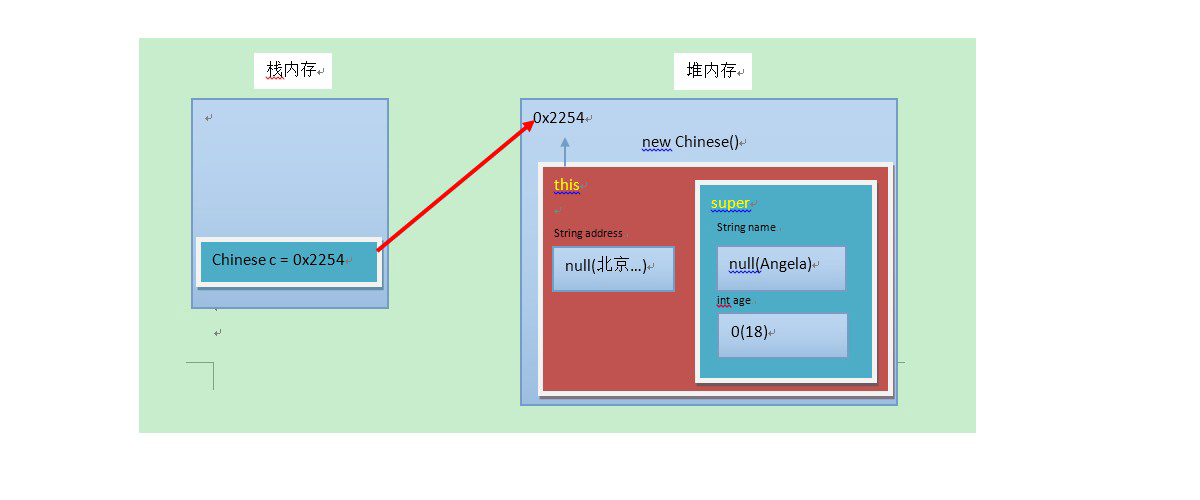### 8.5.2 super和this的含义

• super ：代表父类的存储空间标识(可以理解为父亲的引用)。
• this ：代表当前对象的引用(谁调用就代表谁)。

### 8.5.3super和this的用法

1. 访问成员
``````class Animal {
public void eat() {
System.out.println("animal : eat");
}
}
class Cat extends Animal {
public void eat() {
System.out.println("cat : eat");
}
public void eatTest() {
this.eat(); // this 调用本类的方法
super.eat(); // super 调用父类的方法
}
}
public class ExtendsDemo08 {
public static void main(String[] args) {
Animal a = new Animal();
a.eat();
Cat c = new Cat();
c.eatTest();
}
}

animal : eat
cat : eat
animal : eat``````
1. 访问构造方法
``````this(...) ‐‐ 本类的构造方法
super(...) ‐‐ 父类的构造方法``````

## 8.6 继承的特点

1. Java只支持单继承，不支持多继承。

``````//一个类只能有一个父类，不可以有多个父类。
class C extends A{} //ok
class C extends A，B... //error``````
2. Java支持多层继承(继承体系)。
顶层父类是Object类。所有的类默认继承Object，作为父类。
``````class A{}
class B extends A{}
class C extends B{}``````

1. 子类和父类是一种相对的概念。

## 8.7 抽象类

``````package com.abstractclass;
//这是一个抽象类
/*1.不能直接创建抽象类对象
* 2.必须一个子类继承抽象类
* 3.子类必须实现抽象类所有抽象方法*/
public abstract class Animal {
//    只是一个抽象方法
abstract void eat();
//    这是普通成员方法
public void normalMethod(){};
}``````
``````package com.abstractclass;
//继承抽象类的子类必须重写父类所有的抽象方法。否则，该子类也必须声明为抽象类。最终，必须有子类实现该父类的抽象方法，否则，从最初的父类到最终的子类都不能创建对象，失去意义。
public class Cat extends Animal{
@Override
public void eat(){
System.out.println("猫吃鱼");
}
}``````

``````/*1. 抽象类不能创建对象，如果创建，编译无法通过而报错。只能创建其非抽象子类的对象。

2. 抽象类中，可以有构造方法，是供子类创建对象时，初始化父类成员使用的。

3. 抽象类中，不一定包含抽象方法，但是有抽象方法的类必定是抽象类。

4. 抽象类的子类，必须重写抽象父类中所有的抽象方法，否则，编译无法通过而报错。除非该子类也是抽象类。

package com.abstractclass.demo;

public class DemoMain {
public static void main(String[] args) {
Hasig hasig=new Hasig();
hasig.eat();
hasig.sleep();

Golden golden=new Golden();
golden.eat();
golden.sleep();
}
}``````
``````package com.abstractclass.demo;

public abstract class Animal {

public Animal() {
System.out.println("抽象类构造方法执行");
}

public abstract void eat();
public abstract void sleep();
}
``````
``````package com.abstractclass.demo;

public abstract class Dog extends Animal {
@Override
public void eat() {
System.out.println("狗吃骨头");
}

}``````
``````package com.abstractclass.demo;

public class Golden extends Dog{
@Override
public void sleep() {
System.out.println("金毛睡一天");
}
}``````
``````package com.abstractclass.demo;

public class Hasig extends Dog{

@Override
public void sleep() {
System.out.println("哈士奇不睡觉");
}
}
``````

# 9. 【接口、多态】

## 9.1 接口的基本实现

### 9.1.1 抽象方法的使用

``````public interface LiveAble {
// 定义抽象方法
public abstract void eat();
public abstract void sleep();
}``````

``````public class Animal implements LiveAble {
@Override
public void eat() {
System.out.println("吃东西");
}
@Override
public void sleep() {
System.out.println("晚上睡");
}
}``````

``````public class InterfaceDemo {
public static void main(String[] args) {
// 创建子类对象
Animal a = new Animal();
// 调用实现后的方法
a.eat();
a.sleep();
}
}

### 9.1.2 默认方法的使用

1. 继承默认方法

``````public interface LiveAble {
public default void fly(){
System.out.println("天上飞");
}
}``````

``````public class Animal implements LiveAble {
// 继承，什么都不用写，直接调用
}``````

``````public class InterfaceDemo {
public static void main(String[] args) {
// 创建子类对象
Animal a = new Animal();
// 调用默认方法
a.fly();
}
}

1. 重写默认方法

``````public interface LiveAble {
public default void fly(){
System.out.println("天上飞");
}
}``````

``````public class Animal implements LiveAble {
@Override
public void fly() {
System.out.println("自由自在的飞");
}
}``````

``````public class InterfaceDemo {
public static void main(String[] args) {
// 创建子类对象
Animal a = new Animal();
// 调用默认方法
a.fly();
}
}

### 9.1.3 静态方法的使用

``````public interface LiveAble {
public static void run(){
System.out.println("跑起来~~~");
}
}``````

``````public class Animal implements LiveAble {
// 无法重写静态方法
}``````

``````public class InterfaceDemo {
public static void main(String[] args) {
// Animal.run(); // 【错误】无法继承方法,也无法调用
LiveAble.run(); //
}
}

### 9.1.4 私有方法的使用

• 私有方法：只有默认方法可以调用。
• 私有静态方法：默认方法和静态方法可以调用。

``````public interface LiveAble {
default void func(){
func1();
func2();
}
private void func1(){
System.out.println("跑起来~~~");
}
private void func2(){
System.out.println("跑起来~~~");
}
}``````

``````public class Animal implements LiveAble {
// 继承，什么都不用写，直接调用
}``````

``````public class InterfaceDemo {
public static void main(String[] args) {
// 创建子类对象
Animal a = new Animal();
// 调用默认方法
a.func();/
}
}

## 9.2 接口的多实现

``````class 类名 [extends 父类名] implements 接口名1,接口名2,接口名3... {
// 重写接口中抽象方法【必须】
// 重写接口中默认[ ]： 表示可选操作。方法【不重名时可选】
}
//[ ]： 表示可选操作。``````

### 9.2.1 抽象方法

``````interface A {
public abstract void showA();
public abstract void show();
}
interface B {
public abstract void showB();
public abstract void show();
}``````

``````/*

1. 接口是没有静态代码块或者构造方法的。
2. 一个类的直接父类是唯一的，但是一个类可以同时实现多个接口。

public class MyInterfaceImpl implements MyInterfaceA, MyInterfaceB {
// 覆盖重写所有抽象方法
}
3. 如果实现类所实现的多个接口当中，存在重复的抽象方法，那么只需要覆盖重写一次即可。
4. 如果实现类没有覆盖重写所有接口当中的所有抽象方法，那么实现类就必须是一个抽象类。
5. 如果实现类实现的多个接口当中，存在重复的默认方法，那么实现类一定要对冲突的默认方法进行覆盖重写。
6. 一个类如果直接父类当中的方法，和接口当中的默认方法产生了冲突，优先用父类当中的方法。
*/
public class C implements A,B{
@Override
public void showA() {
System.out.println("showA");
}
@Override
public void showB() {
System.out.println("showB");
}
@Override
public void show() {
System.out.println("show");
}
}``````

### 9.2.2 默认方法

``````interface A {
public default void methodA(){}
public default void method(){}
}
interface B {
public default void methodB(){}
public default void method(){}
}``````

``````public class C implements A,B{
@Override
public void method() {
System.out.println("method");
}
}``````

### 9.3.4 优先级的问题

``````interface A {
public default void methodA(){
System.out.println("AAAAAAAAAAAA");
}
}``````

``````class D {
public void methodA(){
System.out.println("DDDDDDDDDDDD");
}
}``````

``````class C extends D implements A {
// 未重写methodA方法
}``````

``````public class Test {
public static void main(String[] args) {
C c = new C();
c.methodA();
}
}

DDDDDDDDDDDD``````

## 9.3 接口的多继承

``````interface A {
public default void method(){
System.out.println("AAAAAAAAAAAAAAAAAAA");
}
}
interface B {
public default void method(){
System.out.println("BBBBBBBBBBBBBBBBBBB");
}
}``````

``````interface D extends A,B{
@Override
public default void method() {
System.out.println("DDDDDDDDDDDDDD");
}
}``````

## 9.4 其它成员特点

• 接口中，无法定义成员变量，但是可以定义常量，其值不可以改变，默认使用public static final修饰。
• 接口中，没有构造方法，不能创建对象。
• 接口中，没有静态代码块。

## 9.5 多态

• 多态： 是指同一行为，具有多个不同表现形式。

1. 继承或者实现【二选一】
2. 方法的重写【意义体现：不重写，无意义】
3. 父类引用指向子类对象【格式体现】

### 9.5.1 多态的体现

``````父类类型 变量名 = new 子类对象；

``````Fu f = new Zi();
f.method();``````

``````public abstract class Animal {
public abstract void eat();
}``````

``````class Cat extends Animal {
public void eat() {
System.out.println("吃鱼");
}
}
class Dog extends Animal {
public void eat() {
System.out.println("吃骨头");
}
}``````

``````public class Test {
public static void main(String[] args) {
// 多态形式，创建对象
Animal a1 = new Cat();
// 调用的是 Cat 的 eat
a1.eat();
// 多态形式，创建对象
Animal a2 = new Dog();
// 调用的是 Dog 的 eat
a2.eat();
}
}``````

### 9.5.2 多态的好处

``````public abstract class Animal {
public abstract void eat();
}``````

``````class Cat extends Animal {
public void eat() {
System.out.println("吃鱼");
}
}
class Dog extends Animal {
public void eat() {
System.out.println("吃骨头");
}
}``````

``````/*

看new的是谁，就优先用谁，没有则向上找。

*/
public class Test {
public static void main(String[] args) {
// 多态形式，创建对象
Cat c = new Cat();
Dog d = new Dog();
// 调用showCatEat
showCatEat(c);
// 调用showDogEat
showDogEat(d);
/*
以上两个方法, 均可以被showAnimalEat(Animal a)方法所替代
而执行效果一致
*/
showAnimalEat(c);
howAnimalEat(d);
}
public static void showCatEat (Cat c){
c.eat();
}
public static void showDogEat (Dog d){
d.eat();
}
public static void showAnimalEat (Animal a){
a.eat();
}
}``````

## 9.6 引用类型转换

### 9.6.1 向上转型

• 向上转型：多态本身是子类类型向父类类型向上转换的过程，这个过程是默认的。

``````父类类型 变量名 = new 子类类型();

### 9.6.2 向下转型

• 向下转型：父类类型向子类类型向下转换的过程，这个过程是强制的。

``````子类类型 变量名 = (子类类型) 父类变量名;

### 9.6.3 为什么要转型

``````abstract class Animal {
abstract void eat();
}
class Cat extends Animal {
public void eat() {
System.out.println("吃鱼");
}
//cat类特有方法
public void catchMouse() {
System.out.println("抓老鼠");
}
}
class Dog extends Animal {
public void eat() {
System.out.println("吃骨头");
}
//dog类特有方法
public void watchHouse() {
System.out.println("看家");
}
}``````

``````public class Test {
public static void main(String[] args) {
// 向上转型 多态
Animal a = new Cat();
a.eat(); // 调用的是 Cat 的 eat
//a.catchMouse();//编译错误 Animal类中没有此方法
// 向下转型
Cat c = (Cat)a;
c.catchMouse(); // 调用的是 Cat 的 catchMouse
}
}``````

### 9.6.4 转型异常

``````public class Test {
public static void main(String[] args) {
// 向上转型
Animal a = new Cat();
a.eat(); // 调用的是 Cat 的 eat
// 向下转型
Dog d = (Dog)a;
d.watchHouse(); // 调用的是 Dog 的 watchHouse 【运行报错】
}
}``````

``````变量名 instanceof 数据类型

``````public class Test {
public static void main(String[] args) {
// 向上转型
Animal a = new Cat();
a.eat(); // 调用的是 Cat 的 eat
// 向下转型
if (a instanceof Cat){
Cat c = (Cat)a;
c.catchMouse(); // 调用的是 Cat 的 catchMouse
} else if (a instanceof Dog){
Dog d = (Dog)a;
d.watchHouse(); // 调用的是 Dog 的 watchHouse
}
}
}``````

# 10. 【final、权限、内部类、引用类型】

## 10.1 final关键字

• 用于修饰不可改变内容。

• final： 不可改变。可以用于修饰类、方法和变量。
• 类：被修饰的类，不能被继承。
• 方法：被修饰的方法，不能被重写。
• 变量：被修饰的变量，不能被重新赋值。

### 10.1.1 修饰类

``````final class 类名{

}``````

### 10.1.2 修饰方法

``````修饰符 final 返回值类型 方法名(参数列表){
//方法体
}``````

### 10.1.3 修饰变量

#### 10.1.3.1 局部变量——基本类型

``````public class FinalDemo1 {
public static void main(String[] args) {
// 声明变量，使用final修饰
final int a;
// 第一次赋值
a = 10;
// 第二次赋值
a = 20; // 报错,不可重新赋值
// 声明变量，直接赋值，使用final修饰
final int b = 10;
// 第二次赋值
b = 20; // 报错,不可重新赋值
}
}``````

#### 10.1.3.2 局部变量——引用类型

``````public class FinalDemo2 {
public static void main(String[] args) {
// 创建 User 对象
final User u = new User();
// 创建 另一个 User对象
u = new User(); // 报错，指向了新的对象，地址值改变。
// 调用setName方法
u.setName("张三"); // 可以修改
}
}``````

#### 10.1.3.3 成员变量

• 显示初始化；
``````public class User {
final String USERNAME = "张三";
private int age;
}``````
• 构造方法初始化。
``````public class User {
final String USERNAME ;
private int age;
public User(String username, int age) {
this.USERNAME = username;
this.age = age;
}
}``````

## 10.2 权限修饰符

publicprotecteddefailt(空的)private

• 成员变量使用private ，隐藏细节。
• 构造方法使用public ，方便创建对象。
• 成员方法使用public ，方便调用方法。

## 10.3 内部类

• 成员内部类 ：定义在类中方法外的类。

``````class 外部类 {
class 内部类{
}
}``````

• 内部类可以直接访问外部类的成员，包括私有成员。
• 外部类要访问内部类的成员，必须要建立内部类的对象。

``外部类名.内部类名 对象名 = new 外部类型().new 内部类型()；``

``````public class Person {
private boolean live = true;
class Heart {
public void jump() {
// 直接访问外部类成员
if (live) {
System.out.println("心脏在跳动");
} else {
System.out.println("心脏不跳了");
}
}
}
public boolean isLive() {
return live;
}
public void setLive(boolean live) {
this.live = live;
}
}``````

``````public class InnerDemo {
public static void main(String[] args) {
// 创建外部类对象
Person p = new Person();
// 创建内部类对象
Heart heart = p.new Heart();
// 调用内部类方法
heart.jump();
// 调用外部类方法
p.setLive(false);
// 调用内部类方法
heart.jump();
}
}

### 10.3.1 匿名内部类【重点】

• 匿名内部类 ：是内部类的简化写法。它的本质是一个带具体实现的 父类或者父接口的匿名的子类对象。开发中，最常用到的内部类就是匿名内部类了。以接口举例，当你使用一个接口时，似乎得做如下几步操作，
1. 定义子类
2. 重写接口中的方法
3. 创建子类对象
4. 调用重写后的方法

``````new 父类名或者接口名(){
// 方法重写
@Override
public void method() {
// 执行语句
}
};``````

``````public abstract class FlyAble{
public abstract void fly();
}``````

``````public class InnerDemo {
public static void main(String[] args) {
/*
1.等号右边:是匿名内部类，定义并创建该接口的子类对象
2.等号左边:是多态赋值,接口类型引用指向子类对象
*/
FlyAble f = new FlyAble(){
public void fly() {
System.out.println("我飞了~~~");
}
};
//调用 fly方法,执行重写后的方法
f.fly();
}
}``````

``````public class InnerDemo2 {
public static void main(String[] args) {
/*
1.等号右边:定义并创建该接口的子类对象
2.等号左边:是多态,接口类型引用指向子类对象
*/
FlyAble f = new FlyAble(){
public void fly() {
System.out.println("我飞了~~~");
}
};
// 将f传递给showFly方法中
showFly(f);
}
public static void showFly(FlyAble f) {
f.fly();
}
}``````

``````public class InnerDemo3 {
public static void main(String[] args) {
/*
创建匿名内部类,直接传递给showFly(FlyAble f)
*/
showFly( new FlyAble(){
public void fly() {
System.out.println("我飞了~~~");
}
});
}
public static void showFly(FlyAble f) {
f.fly();
}
}``````

## 10.4 引用类型用法总结

### 10.4.1 class作为成员变量

``````package com.innerClass.demo06;

// 游戏当中的英雄角色类
public class Hero {

private String name; // 英雄的名字
private int age; // 英雄的年龄
private Weapon weapon; // 英雄的武器

public Hero() {
}

public Hero(String name, int age, Weapon weapon) {
this.name = name;
this.age = age;
this.weapon = weapon;
}

public void attack() {
System.out.println("年龄为" + age + "的" + name + "用" + weapon.getCode() + "攻击敌方。");
}

public String getName() {
return name;
}

public void setName(String name) {
this.name = name;
}

public int getAge() {
return age;
}

public void setAge(int age) {
this.age = age;
}

public Weapon getWeapon() {
return weapon;
}

public void setWeapon(Weapon weapon) {
this.weapon = weapon;
}
}``````

``````ackage com.innerClass.demo06;

public class Weapon {

private String code; // 武器的代号

public Weapon() {
}

public Weapon(String code) {
this.code = code;
}

public String getCode() {
return code;
}

public void setCode(String code) {
this.code = code;
}
}``````

``````package com.innerClass.demo06;

import java.util.Scanner;

public class DemoMain {

public static void main(String[] args) {
// 创建一个英雄角色
Hero hero = new Hero();
// 为英雄起一个名字，并且设置年龄
System.out.println("英雄名字：");
hero.setName(new Scanner(System.in).next());
System.out.println("英雄年龄：");
hero.setAge(new Scanner(System.in).nextInt());

// 创建一个武器对象
Weapon weapon = new Weapon("AK-47");
// 为英雄配备武器
hero.setWeapon(weapon);

// 年龄为20的盖伦用多兰剑攻击敌方。
hero.attack();
}

}``````

### 10.4.2 interface作为成员变量

``````// 法术攻击
public interface FaShuSkill {
public abstract void faShuAttack();
}``````

``````public class Role {
FaShuSkill fs;//接口作为成员变量
public void setFaShuSkill(FaShuSkill fs) {
this.fs = fs;
}
// 法术攻击
public void faShuSkillAttack(){
System.out.print("发动法术攻击:");
fs.faShuAttack();
System.out.println("攻击完毕");
}
}``````

``````public class Test {
public static void main(String[] args) {
// 创建游戏角色
Role role = new Role();
// 设置角色法术技能
role.setFaShuSkill(new FaShuSkill() {
@Override
public void faShuAttack() {
System.out.println("纵横天下");
}
});
// 发动法术攻击
role.faShuSkillAttack();
// 更换技能
role.setFaShuSkill(new FaShuSkill() {
@Override
public void faShuAttack() {
System.out.println("逆转乾坤");
}
});
// 发动法术攻击
role.faShuSkillAttack();
}
}

### 10.4.3 interface作为方法参数和返回值类型

``````public static List<Integer> getEvenNum(List<Integer> list) {
// 创建保存偶数的集合
ArrayList<Integer> evenList = new ArrayList<>();
// 遍历集合list,判断元素为偶数,就添加到evenList中
for (int i = 0; i < list.size(); i++) {
Integer integer = list.get(i);
if (integer % 2 == 0) {
evenList.add(integer);
}
}
/*
返回偶数集合
因为getEvenNum
因为getEvenNum方法的返回值类型是List,而ArrayList是List的子类,
所以evenList可以返回
*/
return evenList;
}``````

``````public class Test {
public static void main(String[] args) {
// 创建ArrayList集合,并添加数字
ArrayList<Integer> srcList = new ArrayList<>();
for (int i = 0; i < 10; i++) {
srcList.add(i);
}
/*
获取偶数集合
因为getEvenNum方法的参数是List,而ArrayList是List的子类,
所以srcList可以传递
*/
List list = getEvenNum(srcList);
System.out.println(list);
}
}``````

### 发表评论email
web

### 全部评论 (共 2 条评论)2021-01-03 09:23
写得非常好！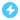2021-01-03 18:00
@Paul୧(๑•̀⌄•́๑)૭